# 2021-09-17AI 同时被 2 个专栏收录2 篇文章 0 订阅

# 排序损失-Rating Loss-举例说明

ℓ rank  = 1 m + m − ∑ x + ∈ D + ∑ x − ∈ D − ( I ( f ( x + ) < f ( x − ) ) + 1 2 I ( f ( x + ) = f ( x − ) ) ) \ell_{\text {rank }}=\frac{1}{m^{+} m^{-}} \sum_{\boldsymbol{x}^{+} \in D^{+}} \sum_{\boldsymbol{x}^{-} \in D^{-}}\left(\mathbb{I}\left(f\left(\boldsymbol{x}^{+}\right)<f\left(\boldsymbol{x}^{-}\right)\right)+\frac{1}{2} \mathbb{I}\left(f\left(\boldsymbol{x}^{+}\right)=f\left(\boldsymbol{x}^{-}\right)\right)\right)

## 1 定义

### 1.1 ROC图：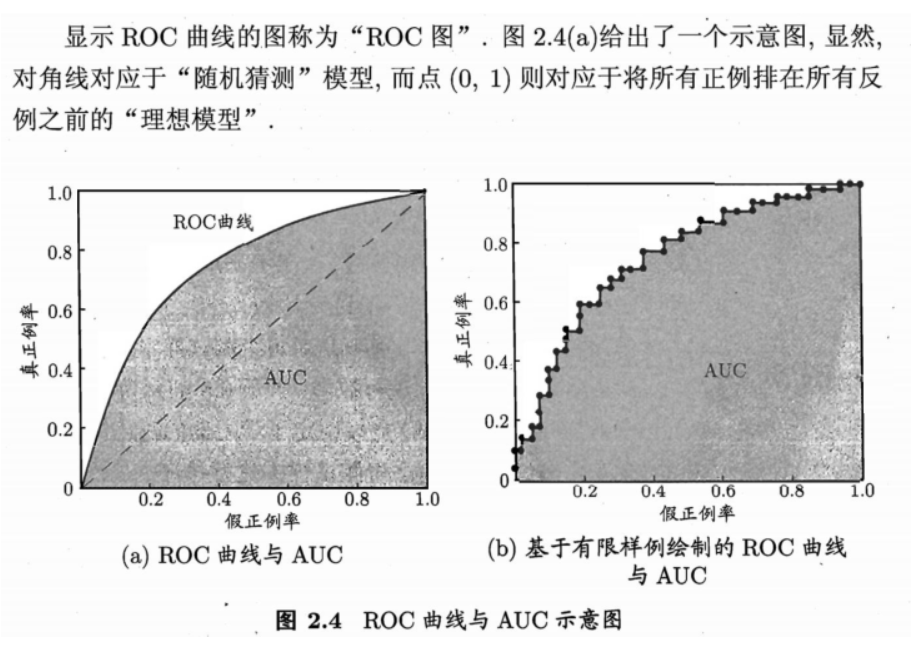### 1.2 AUC指标

• 从定义可知，AUC可通过对ROC曲线下各部分的面积求和而得.
• 假定ROC曲线是由坐标为 { ( x 1 , y 1 ) , ( x 2 , y 2 ) , … , ( x m , y m ) } \left\{\left(x_{1}, y_{1}\right),\left(x_{2}, y_{2}\right), \ldots,\left(x_{m}, y_{m}\right)\right\} 的点按序连接而形成 ( x 1 = 0 , x m = 1 ) \left(x_{1}=0, x_{m}=1\right) , 参见周志华西瓜书图2.4(b)，则AUC可估算为【周志华机器学习西瓜书公式(2.20)】

A U C = 1 2 ∑ i = 1 m − 1 ( x i + 1 − x i ) ⋅ ( y i + y i + 1 ) \mathrm{AUC}=\frac{1}{2} \sum_{i=1}^{m-1}\left(x_{i+1}-x_{i}\right) \cdot\left(y_{i}+y_{i+1}\right)

### 1.3 排序误差

• 形式化地看，AUC考虑的是样本预测的排序质量，因此它与排序误差有紧密联系.
• 给定 m + m^+ 个正例和 m − m^- 个反例，令 D + D^+ D − D^- 分别表示正、反例集合，
• 则排序“损失”(loss)定义为【周志华机器学习西瓜书公式(2.21)】

ℓ rank  = 1 m + m − ∑ x + ∈ D + ∑ x − ∈ D − ( I ( f ( x + ) < f ( x − ) ) + 1 2 I ( f ( x + ) = f ( x − ) ) ) \ell_{\text {rank }}=\frac{1}{m^{+} m^{-}} \sum_{\boldsymbol{x}^{+} \in D^{+}} \sum_{\boldsymbol{x}^{-} \in D^{-}}\left(\mathbb{I}\left(f\left(\boldsymbol{x}^{+}\right)<f\left(\boldsymbol{x}^{-}\right)\right)+\frac{1}{2} \mathbb{I}\left(f\left(\boldsymbol{x}^{+}\right)=f\left(\boldsymbol{x}^{-}\right)\right)\right)

• i) 这里 I ( ⋅ ) \mathbb{I}(\cdot) 是指示函数，若 ⋅ \cdot 为真则取值1, 否则取值0.

• ii) 公式解释：

• 考虑每一对正、反例，若正例的预测值小于反例，则记 1 个“罚分”
• 若相等，则记 0.5 个“罚分”.

### 1.4 排序误差和AUC之间的关系

• ℓ rank  \ell_{\text {rank }} 对应的是ROC曲线之上的面积
• 一个正例在ROC曲线上对应标记点的坐标为 ( x , y ) (x,y) ，则 x x 恰是排序在其之前的反例所占的比例，即假正例率.
• 因此有【周志华机器学习西瓜书公式(2.22)】

A U C = 1 − ℓ rank AUC=1-\ell_{\text{rank}}

## 2 举例计算 ℓ r a n k \ell_{rank}

• 下面这个例子的目标是：识别手写体数字5

• 共12个对象，6个正例对象，6个反例对象

• 根据某种算法排序如下（判定为数字5的概率，由右至左递减，即 p 12 < p 11 < ⋯ < p 2 < p 1 p_{12}< p_{11}< \cdots< p_{2}< p_{1} ）：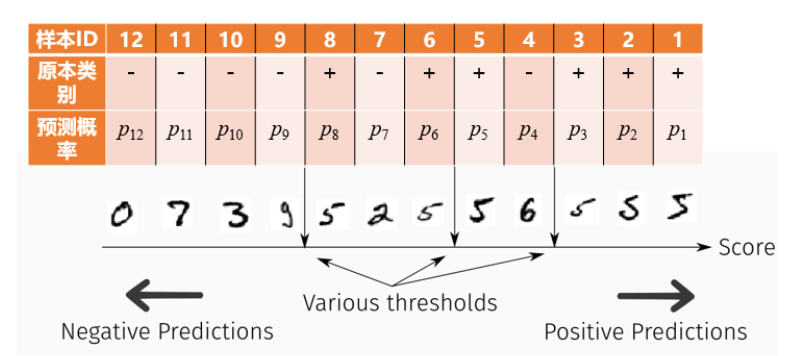• 计算该例的 ℓ r a n k \ell_{rank}

• 公式为: ℓ rank  = 1 m + m − ∑ x + ∈ D + ∑ x − ∈ D − ( I ( f ( x + ) < f ( x − ) ) + 1 2 I ( f ( x + ) = f ( x − ) ) ) \ell_{\text {rank }}=\frac{1}{m^{+} m^{-}} \sum_{\boldsymbol{x}^{+} \in D^{+}} \sum_{\boldsymbol{x}^{-} \in D^{-}}\left(\mathbb{I}\left(f\left(\boldsymbol{x}^{+}\right)<f\left(\boldsymbol{x}^{-}\right)\right)+\frac{1}{2} \mathbb{I}\left(f\left(\boldsymbol{x}^{+}\right)=f\left(\boldsymbol{x}^{-}\right)\right)\right)

• 这里的各个参数的值为：
• m + = m − = 6 m^+=m^-=6
• x + ∈ D + \boldsymbol{x}^{+}\in D^{+} 的样本ID为 { 8 , 6 , 5 , 3 , 2 , 1 } \{8,6,5,3,2,1\} ，对应的 f ( x + ) f\left(\boldsymbol{x}^{+}\right) 值为 { p 8 , p 6 , p 5 , p 3 , p 2 , p 1 } \{p_8,p_6,p_5,p_3,p_2,p_1\} .
• x − ∈ D − \boldsymbol{x}^{-}\in D^{-} 的样本ID为 { 12 , 11 , 10 , 9 , 7 , 4 } \{12,11,10,9,7,4\} ，对应的 f ( x + ) f\left(\boldsymbol{x}^{+}\right) 值为 { p 12 , p 11 , p 10 , p 9 , p 7 , p 4 } \{p_{12},p_{11},p_{10},p_{9},p_{7},p_{4}\} .
• 从左到右
• 对==所有 x − ∈ D − \boldsymbol{x}^{-}\in D^{-} ==计算
• 12号样本是一个 x − \boldsymbol{x}^{-} 所有 x + ∈ D + \boldsymbol{x}^{+}\in D^{+}
• 其预测出的概率值 p 12 p_{12}
• 小于任何一个 x + ∈ D + \boldsymbol{x}^{+}\in D^{+} 的预测概率值 { p 8 , p 6 , p 5 , p 3 , p 2 , p 1 } \{p_8,p_6,p_5,p_3,p_2,p_1\}
• 因此对于所有 x + ∈ D + \boldsymbol{x}^{+}\in D^{+}
• 指示函数 I ( f ( x + ) < f ( x − ) ) \mathbb{I}\left(f\left(\boldsymbol{x}^{+}\right)<f\left(\boldsymbol{x}^{-}\right)\right) I ( f ( x + ) = f ( x − ) ) \mathbb{I}\left(f\left(\boldsymbol{x}^{+}\right)=f\left(\boldsymbol{x}^{-}\right)\right) 的值均为0
• 则根据12号样本计算的 ℓ r a n k \ell_{rank} 的一部分值为0.
• 11、10、9号样本与12号样本情况类似
• 则根据11、10、9号样本计算的 ℓ r a n k \ell_{rank} 的那些部分值为0.
• 7号样本是一个 x − \boldsymbol{x}^{-} ，对于所有 x + ∈ D + \boldsymbol{x}^{+}\in D^{+}
• 其预测出的概率值 p 7 p_{7} 满足
• p 7 < p 6 , p 5 , p 3 , p 2 , p 1 p_{7}<p_6,p_5,p_3,p_2,p_1
• 但是 p 7 > p 8 p_7>p_8
• 因此：
• 对于 { 6 , 5 , 3 , 2 , 1 } \{6,5,3,2,1\} 号样本，指示函数 I ( f ( x + ) < f ( x − ) ) \mathbb{I}\left(f\left(\boldsymbol{x}^{+}\right)<f\left(\boldsymbol{x}^{-}\right)\right) I ( f ( x + ) = f ( x − ) ) \mathbb{I}\left(f\left(\boldsymbol{x}^{+}\right)=f\left(\boldsymbol{x}^{-}\right)\right) 的值为0.
• 对于8号样本，指示函数 I ( f ( x + ) < f ( x − ) ) = 1 \mathbb{I}\left(f\left(\boldsymbol{x}^{+}\right)<f\left(\boldsymbol{x}^{-}\right)\right)=1 , I ( f ( x + ) = f ( x − ) ) \mathbb{I}\left(f\left(\boldsymbol{x}^{+}\right)=f\left(\boldsymbol{x}^{-}\right)\right) 的值为0.
• 又因为 m + = m − = 6 m^+=m^-=6 ，则根据8号样本计算的 ℓ r a n k \ell_{rank} 的一部分值为 1 6 × 6 ⋅ 1 = 1 36 \dfrac{1}{6\times 6}\cdot 1 =\dfrac{1}{36} .
• 4号样本是一个 x − \boldsymbol{x}^{-} ，对于所有 x + ∈ D + \boldsymbol{x}^{+}\in D^{+}
• 其预测出的概率值 p 4 p_{4} 满足
• p 4 < p 3 , p 2 , p 1 p_{4}<p_3,p_2,p_1
• 但是 p 4 > p 8 , p 6 , p 5 p_4>p_8,p_6,p_5
• 因此：
• 对于 { 3 , 2 , 1 } \{3,2,1\} 号样本，指示函数 I ( f ( x + ) < f ( x − ) ) \mathbb{I}\left(f\left(\boldsymbol{x}^{+}\right)<f\left(\boldsymbol{x}^{-}\right)\right) I ( f ( x + ) = f ( x − ) ) \mathbb{I}\left(f\left(\boldsymbol{x}^{+}\right)=f\left(\boldsymbol{x}^{-}\right)\right) 的值为0.
• 对于 { 8 , 6 , 5 } \{8,6,5\} 号样本，指示函数 I ( f ( x + ) < f ( x − ) ) = 1 \mathbb{I}\left(f\left(\boldsymbol{x}^{+}\right)<f\left(\boldsymbol{x}^{-}\right)\right)=1 , I ( f ( x + ) = f ( x − ) ) \mathbb{I}\left(f\left(\boldsymbol{x}^{+}\right)=f\left(\boldsymbol{x}^{-}\right)\right) 的值为0.
• 又因为 m + = m − = 6 m^+=m^-=6 ，则根据4号样本计算的 ℓ r a n k \ell_{rank} 的一部分值为 1 6 × 6 ⋅ 3 = 3 36 \dfrac{1}{6\times 6}\cdot 3 =\dfrac{3}{36} .
• 综上所述， ℓ r a n k = 0 + 0 + 0 + 0 + 1 36 + 3 36 = 4 36 \ell_{rank}=0+0+0+0+\dfrac{1}{36}+\dfrac{3}{36}=\dfrac{4}{36}
• 该例仅考虑了预测概率严格递减的情况，即 p 12 < p 11 < ⋯ < p 2 < p 1 p_{12}< p_{11}< \cdots< p_{2}< p_{1} 的情况，如果是 p 12 ≤ p 11 ≤ ⋯ ≤ p 2 ≤ p 1 p_{12}\leq p_{11}\leq \cdots\leq p_{2}\leq p_{1} ，遇到有等号的情况会导致某些 x − \boldsymbol{x}^{-} x + \boldsymbol{x}^{+} 对应的指示函数 I ( f ( x + ) = f ( x − ) ) = 1 \mathbb{I}\left(f\left(\boldsymbol{x}^{+}\right)=f\left(\boldsymbol{x}^{-}\right)\right)=1 .

## 3 举例画ROC、图示说明AUC

• 同样上面的案例，分别选取阈值为 { 1 , p 1 , p 2 , p 3 , p 4 , p 5 , p 6 , p 7 , p 8 , p 9 , p 10 , p 11 , p 12 , 0 } \{1,p_1,p_2,p_3,p_4,p_5,p_6,p_7,p_8,p_9,p_{10},p_{11},p_{12},0\} 计算ROC曲线的描点，

• 阈值 =1，
• 预测情况
• 所有值均预测为负，
• 则计算得：
• TP=FP=0，TPR=FPR=0/6=0
• ⇒ \Rightarrow 描点（0,0）
• 阈值 = p 1 p_1
• 预测情况
• 仅有1号样本被预测为正，其他样本预测为负，
• 1号样本本就是正的，
• 则计算得
• TP=1，TPR = 1/6,
• FP=0，FPR=0/6=0，
• ⇒ \Rightarrow 描点（0,1/6）
• 阈值 = p 2 p_2
• 预测情况
• 第1、2号样本被预测为正，其他样本预测为负，
• 1、2号样本就是为正
• 计算得
• TP=2，TPR = 2/6,
• FP=0，FPR=0/6=0，
• ⇒ \Rightarrow 描点（0,2/6）
• 阈值 = p 3 p_3
• 预测情况：
• 第1、2、3号样本被预测为正，其他样本预测为负
• 第1、2、3号，三个样本本身为正
• 则计算得：
• TP=3，TPR = 3/6,
• FP=0，FPR=0/6=0，
• ⇒ \Rightarrow 描点（0,3/6）
• 阈值 = p 4 p_4
• 预测情况：
• 第1、2、3、4号样本被预测为正，其他样本预测为负
• 第1、2、3号，三个样本本身为正，4号样本原本为负
• 则计算得：
• TP=3，TPR = 3/6,
• FP=1，FPR=1/6，
• ⇒ \Rightarrow 描点（1/6,3/6）
• 阈值 = p 5 p_5
• 预测情况
• 第1、2、3、4、5号样本被预测为正，其他样本预测为负，
• 第1、2、3、5这四个样本原本为正，4号样本原本为负
• 则计算得
• TP=4，TPR = 4/6,
• 则 FP=1，FPR=1/6，
• ⇒ \Rightarrow 描点（1/6,4/6）
• 阈值 = p 6 p_6
• 预测情况
• 第1、2、3、4、5、6号样本被预测为正，其他样本预测为负，
• 第1、2、3、5、6，这5个样本原本为正，4号样本原本为负
• 则计算得
• TP=,5，TPR = 5/6,
• 则 FP=1，FPR=1/6，
• ⇒ \Rightarrow 描点（1/6,5/6）
• 阈值 = p 7 p_7
• 预测情况
• 第1、2、3、4、5、6、7号样本被预测为正，其他样本预测为负，
• 第1、2、3、5、6，这5个样本原本为正，4、7号，2个样本原本为负
• 则计算得
• TP=,5，TPR = 5/6,
• 则 FP=2，FPR=2/6，
• ⇒ \Rightarrow 描点（2/6,5/6）
• 其他类似，还可
• ⇒ \Rightarrow 描点 (2/6,6/6),
• ⇒ \Rightarrow 描点(3/6,6/6),
• ⇒ \Rightarrow 描点(4/6,6/6),
• ⇒ \Rightarrow 描点(6/6,6/6),
• ⇒ \Rightarrow 描点(6/6,6/6)
• 根据描点作图

•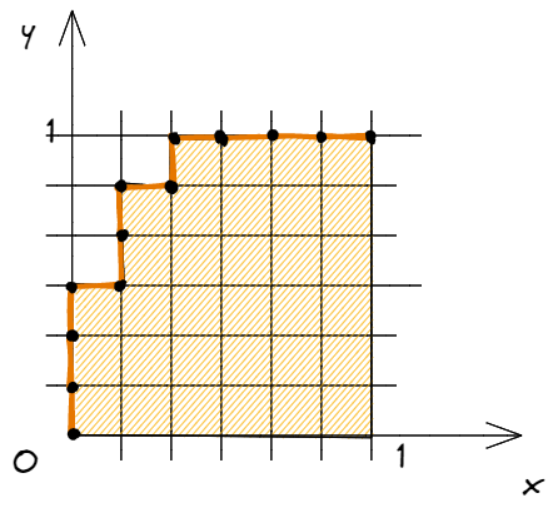• 描点过程中ROC点的走势变化，变换一次阈值时（由高逐次降低，每降低一次），可能发生的情况：

•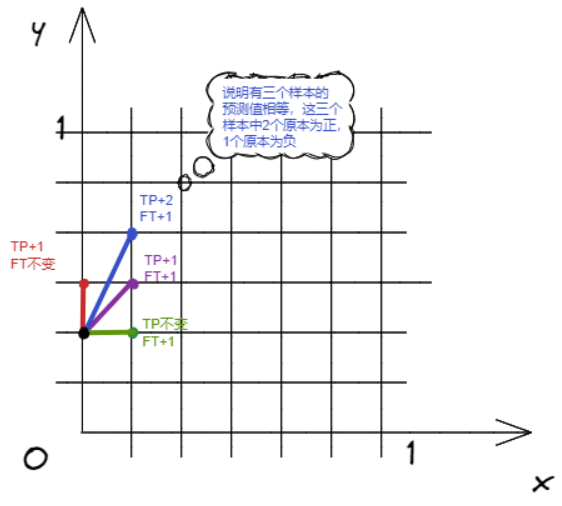• 图示的四种情况说明：

• 1）TP+1，FP不变（红色）
• 某个本来为正的样本，预测变成正的了，即 TP+1，
• 本来为负的所有样本，预测值全部都没有发生变化
• ⇐ \Leftarrow 此时：该阈值为某个正样本的预测概率值
• 2）TP不变，FP+1（绿色）
• 本来为正的所有样本，预测值全部都没有发生变化
• 某个本来为负的样本，预测变成正的了，即 FP+1，
• ⇐ \Leftarrow 此时：该阈值为某个负样本的预测概率值
• 3）TP+1，FP+1（紫色）
• 某个本来为正的样本，预测变成正的了，即 TP+1，
• 某个本来为负的样本，预测变成正的了，即 FP+1，
• ⇐ \Leftarrow 此时：有一个正样本和一个负样本的概率预测值都是该阈值
• 4）TP+2，PF+3（类似蓝色，蓝色为TP+1，PF+2）
• 某2个本来为正的样本，预测变成正的了，即 TP+2，
• 某3个本来为负的样本，预测变成正的了，即 FP+3，
• ⇐ \Leftarrow 此时：有2个正样本和3个负样本的概率预测值相等，都是该阈值（这里 2,3 也可以换为其他正整数，该情况其实可以囊括前三种情况）
• 【周志华机器学习西瓜书公式(2.20)】提到过AUC可估算为

A U C = 1 2 ∑ i = 1 m − 1 ( x i + 1 − x i ) ⋅ ( y i + y i + 1 ) \mathrm{AUC}=\frac{1}{2} \sum_{i=1}^{m-1}\left(x_{i+1}-x_{i}\right) \cdot\left(y_{i}+y_{i+1}\right)

• 可以理解为求梯形面积 A U C = ∑ i = 1 m − 1 1 2 ( x i + 1 − x i ) ⋅ ( y i + y i + 1 ) = ∑ i = 1 m − 1 ( x i + 1 − x i ) ⋅ 1 2 ( y i + y i + 1 ) \mathrm{AUC}=\sum_{i=1}^{m-1} \frac{1}{2}\left(x_{i+1}-x_{i}\right) \cdot \left(y_{i}+y_{i+1}\right)=\sum_{i=1}^{m-1}\left(x_{i+1}-x_{i}\right) \cdot \frac{1}{2} \left(y_{i}+y_{i+1}\right)
• 这里 x i + 1 − x i x_{i+1}-x_i 是梯形的高，上下底的高度分别为 y i , y i + 1 y_{i}, y_{i+1} .
• 如图：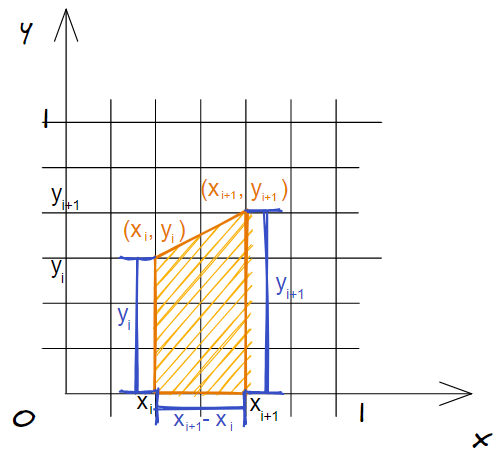## 4 排序误差 ℓ rank \ell_{\text{rank}} 和AUC的关系图示

• 排序误差+AUC=1，即 A U C = 1 − ℓ rank AUC=1-\ell_{\text{rank}} .

•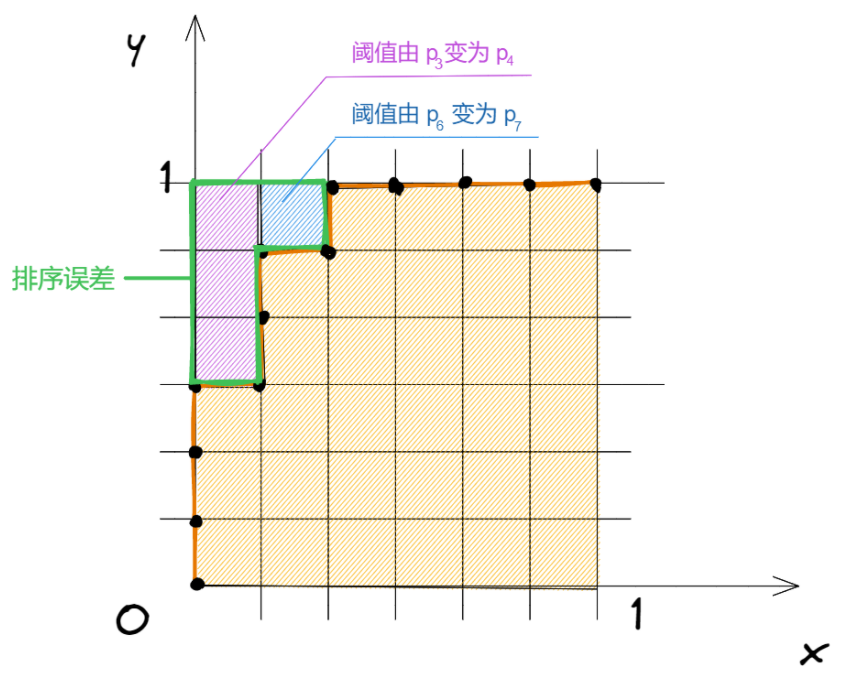09-17809-0240
09-1515
09-1613
06-09145
09-0147
09-0135
09-1427
09-051162
09-17820
09-0750点击重新获取扫码支付1.余额是钱包充值的虚拟货币，按照1:1的比例进行支付金额的抵扣。
2.余额无法直接购买下载，可以购买VIP、C币套餐、付费专栏及课程。余额充值